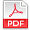## Scientific Review

Online ISSN: 2412-2599
Print ISSN: 2413-8835

# Archives

### Volume 1 Number 7 December 2015

#### Critical Overview of Some Pumping Test Analysis EquationsPDF

Authors: Akudinobi B.E.B. ; Egboka B.C.E. ; Moore C. ; Obiadi I.I.
Pages: 135-142
Abstract
Possible methods of providing further (and perhaps better) alternative solutions for the exponential integral of aquifer parameter evaluation are investigated. Three known mathematical methods of approach (comprising self-similar, separable variable and travelling wave) are applied, providing three relevant solutions. Further analysis of the self-similar solution reveals that this provides an alternative solution involving normal graph of drawdown versus the measurement intervals. The geomathematical relevance of this method is assessed using data from aquifers from two chronologically different hydrogeological units – the Ajalli Sandstone and Ogwashi-Asaba Formation. The results indicate good functional relationship with satisfactory transmissivity values..

#### The Ancient-Greek Special Problems, as the Quantization Moulds of SpacesPDF

Authors: Markos Georgallides
Pages: 113-134
Abstract
The Special  Problems of  E-geometry consist the , Mould Quantization , of  Euclidean Geometry in it , to become → Monad , through mould of  Space –Anti-space in itself , which is the material dipole in inner monad Structure as the Electromagnetic cycloidal field → Linearly , through mould of  Parallel Theorem  [44-45], which are the equal distances between points of parallel  and line  →  In Plane ,  through mould of  Squaring the circle   , where  the two equal and perpendicular monads consist  a Plane acquiring  the common  Plane-meter  →  and in Space (volume)  , through mould of  the Duplication of  the Cube   , where any  two Unequal  perpendicular monads  acquire the common  Space-meter  to be twice each other , as analytically  all methods are proved and explained . [39-41]. The Unification of Space and Energy becomes through [STPL] Geometrical Mould Mechanism of Elements , the minimum Energy-Quanta , In monads → Particles , Anti-particles , Bosons , Gravity –Force , Gravity -Field , Photons , Dark Matter , and Dark-Energy ,consisting Material Dipoles in inner monad  Structures  i.e. the Electromagnetic Cycloidal  Field of  monads. Euclid’s elements consist of assuming a small set of intuitively appealing axioms , proving many other propositions . Because nobody until  succeeded to prove the parallel postulate by means of pure geometric logic , many self-consistent non - Euclidean geometries have been discovered , based on Definitions , Axioms or Postulates , in order that none of them contradicts any of the other postulates . In   the only Space-Energy geometry is Euclidean , agreeing with the Physical reality , on  unit AB = Segment which is The Electromagnetic field of the Quantized on AB Energy Space Vector , on the contrary to the General relativity of Space-time which is based on the rays of the non-Euclidean geometries to the limited velocity of light and Planck`s cavity . Euclidean geometry elucidated the definitions of geometry-content ,{ for Point , Segment , Straight Line , Plane , Volume, Space [S] , Anti-space [AS] , Sub-space [SS] , Cave, Space-Anti-Space Mechanism of the Six-Triple-Points-Line , that produces and transfers Points of Spaces , Anti-Spaces and Sub-Spaces in a Common Inertial Sub-Space and a cylinder ,Gravity field [MFMF] , Particles } and describes the Space-Energy beyond Plank´s length level [ Gravity Length 3,969.10 ̄ 62 m ] , reaching the Point =  L_( v) =  e^(i.(Nπ/2)b=10  ͞ N= - ∞) m = 0 m , which is nothing  and zero space .[43-46] -The Geometrical solution of  the Special Problems is now presented .